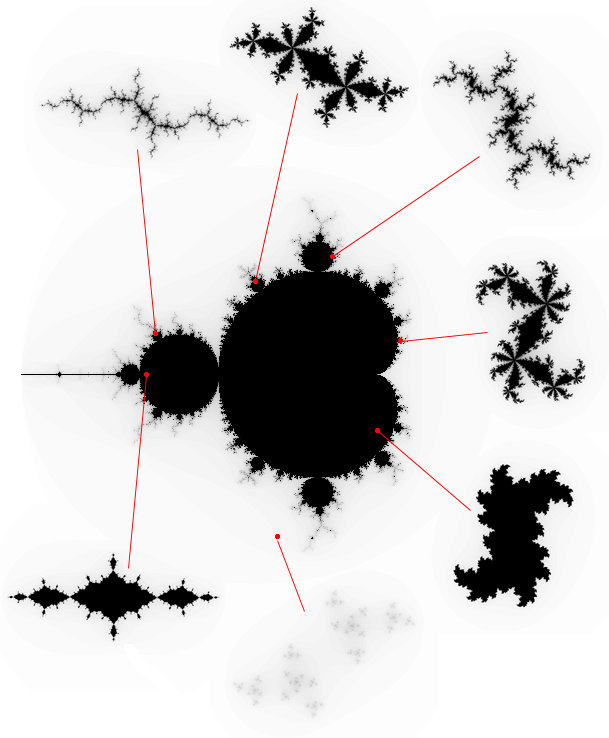﻿ Introduction to the Mandelbrot and Julia sets

# Introduction to the Mandelbrot

written by Ruben van Nieuwpoort

The Mandelbrot sets is named after Benoit Mandelbrot. It is mostly know for the pretty pictures it can produce, while being relatively easy to render.

## Definition

The Mandelbrot is a subset of the complex numbers. It consists of the complex numbers $c$ for which the magnitude of the elements in the sequence
$z_0 = 0 \\z_{n + 1} = z_n^2 + c$
remains bounded.

Since every complex number has both a real and an imaginary part, the space of complex numbers is two-dimensional and maps nicely to a computer screen. By convention, the y-axis corresponds to the imaginary part of $c$, and the x-axis to the real part.

Plots of the set are obtained by seeing if the magnitude of the complex iterands is bigger than some predefined escape radius. It can be shown that a radius of 2 is big enough, in the sense that if we have $|z_n| > 2$ for some $n$, then $\lim_{n \rightarrow \infty} |z_n| = \infty$.

If the magnitude of the iterand is not bigger than the escape radius after some predefined maximum number of iterations, the point is considered to be in the Mandelbrot set. So, plots are merely an approximation.A plot of the Mandelbrot set from Wikipedia, along with the real and imaginary axes.

This plot doesn’t really do justice to the beauty of the Mandelbrot set. First, it is monochrome. More interesting pictures can be obtained by using a color that is based on the iteration number $n$ in which the magnitude of the iterand first exceeded the escape radius.Plot of the Mandelbrot set using the escape time algorithm. This image was released into the public domain by Michael Bradshad.

It looks even better when you use a smooth coloring scheme, which approximates the iteration count with a continuous function, which gives a much smoother appearance.Plot of the Mandelbrot set, using the normalized iteration count algorithm. This image was released into the public domain by Michael Bradshad.

Second, the most interesting pictures of the Mandelbrot set can be obtained by zooming in on regions near the border. Sometimes, regions are similar to the whole Mandelbrot set.Self-similar regions of the Mandelbrot set. This image was released into the public domain by Ishaan Gulrajani.

## Pseudocode to plot the Mandelbrot set

This pseudocode plots a monochrome plot of the Mandelbrot set.

center_r = -0.5
center_i = 0
scale = 0.005
maxiter = 255
for y = 0 to height
for x = 0 to width
escape = false
n = 0
c_r = center_r + (x - width / 2) * scale
c_i = center_i + (y - height / 2) * scale
while n < maxiter and not(escape):
n = n + 1
z_r_new = z_r * z_r - z_i * z_i + c_r
z_i_new = 2 * z_r * z_i + c_i
z_r = z_r_new
z_i = z_i_new
if z_r * z_r + z_i * z_i > 4 then escape = true
if escape then plot(x, y, rgb(0, 0, 0))
else plot(x, y, rgb(255, 255, 255))


## Julia sets

The Julia set of the map $z \mapsto z^2 + c$ is related to the Mandelbrot set. The formal definition of the Julia set is a bit tricky (by this I mean that I don’t understand it at the time of writing…). For the Mandelbrot set, it can be obtained as the set of complex numbers $z_0$ for which the sequence
$z_{n + 1} = z_n^2 + c$
is bounded. Since the sequence does not only depend on $z_0$, but also on $c$, we can obtain Julia sets for various points. The most interesting points lie on the border of the Mandelbrot set.Points in the Mandelbrot set and their corresponding Julia sets. Image generated by Paul Bourke and obtained from his article about the Julia set. Used with permission.

It is interesting to note that there is some similarity between some regions in the Mandelbrot set, and some of the Julia sets of the mapping $z \mapsto z^2 + c$.Top: section of the Mandelbrot set. Bottom: Julia set. This image was released in the public domain by the wikipedia user “Simpsons contributor”.

The Buddhabrot is strongly related to the Mandelbrot fractal. The plot is obtained by plotting every iterand $z_n$. This article by Paul Bourke is a nice introduction, just like this article by Melinda Green.